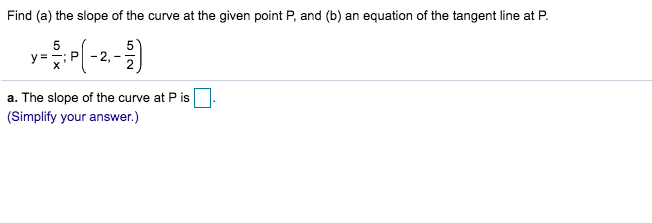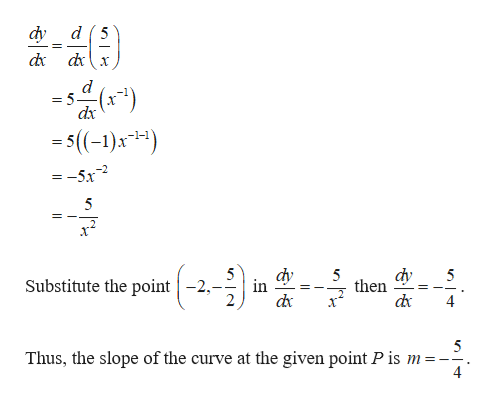Find (a) the slope of the curve at the given point P, and (b) an equation of the tangent line at P55Py=Xa. The slope of the curve at P is(Simplify your answer.)

Question

Can i get help with this problem step by step?help_outlineImage TranscriptioncloseFind (a) the slope of the curve at the given point P, and (b) an equation of the tangent line at P 5 5 P y= X a. The slope of the curve at P is (Simplify your answer.) fullscreen
Step 1

(a)

The given equation is y = 5/x.

Differentiate the equation y = 5/...help_outlineImage Transcriptionclosed 5 dy dx - 5 dx 5((-1)x1) = =-5x dy in 5 dy then 5 Substitute the point -2, dx 4 5 Thus, the slope of the curve at the given point P is m 4 fullscreen

Want to see the full answer?

See Solution

Want to see this answer and more?

Our solutions are written by experts, many with advanced degrees, and available 24/7

See Solution
Tagged in

Calculus